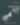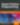# Fun and Functional Programming in PHP with Macros

PHP

Share:

#### Free JavaScript Book!Write powerful, clean and maintainable JavaScript.

RRP \$11.95

I was so excited about my previous article about PHP macros, that I thought it would be fun for us to explore the intersection of macros and functional programming.

PHP is already full of functions, with object oriented patterns emerging relatively late in its life. Still, PHP’s functions can be cumbersome, especially when combined with variable scope rules…Consider the following ES6 (JavaScript) code:

``````let languages = [
{"name": "JavaScript"},
{"name": "PHP"},
{"name": "Ruby"},
];

const prefix = "language: ";

console.log(
languages.map(
language => prefix + language.name
)
);
``````

In this example, we see `languages` defined as a list of programming languages. Each programming language is combined with a constant prefix and the resulting array is logged to the console.

This is about as much JavaScript as we’re going to see. If you’d like to learn more ES6, check out the documentation for BabelJS.

Compare this to similar PHP code:

``````\$languages = [
["name" => "JavaScript"],
["name" => "PHP"],
["name" => "Ruby"],
];

\$prefix = "language: ";

var_dump(
array_map(function(\$language) use (\$prefix) {
return \$prefix . \$language;
}, \$languages);
);
``````

It’s not significantly more code, but it isn’t as clear or idiomatic as the JavaScript alternative. I often miss JavaScript’s expressive, functional syntax, when I’m building PHP things. I want to try and win back some of that expressive syntax!

## Getting Started

As in the previous article, we’re going to use Márcio Almada’s Yay library. We can install it with:

``````composer require yay/yay:*
``````

We’ll put our Yay code (very similar to PHP, but with macro support) in files ending in `.yphp` and compile them to regular PHP with:

``````vendor/bin/yay before.yphp > after.php
``````

## A Simple Solution

The first thing I want to try is mapping over a collection of things, with syntax more similar to JavaScript. I want something like:

``````\$list->map(function(\$item) {
return strtoupper(\$item);
});
``````

We can already do that, but it requires creating a special PHP class. We can’t use normal array functions on this special class, so the class also needs to have methods to convert to and from native PHP arrays.``````macro {
T_VARIABLE·A->map(···expression)
} >> {
array_map(···expression, T_VARIABLE·A)
}
``````

This macro will match anything that looks like a variable, followed by `->map(...)`; and convert it to the `array_map(...)` alternative. So, if we feed it this code:

``````\$languages = [
["name" => "JavaScript"],
["name" => "PHP"],
["name" => "Ruby"],
];

var_dump(
\$languages->map(function(\$language) {
return strtoupper(\$language["name"]);
})
);
``````

…it will compile to:

``````\$languages = [
["name" => "JavaScript"],
["name" => "PHP"],
["name" => "Ruby"],
];

var_dump(
array_map(function(\$language) {
return strtoupper(\$language["name"]);
}, \$languages)
);
``````

If you haven’t already, now is a good time to read the previous article, which explains how this code works. You definitely shouldn’t try the following examples if you’re not comfortable with what you’ve seen so far…

## A Complex Solution

We’re off to a good start! There’s still a significant difference between JavaScript and PHP: variable scope. If we wanted to use that prefix, we would have to bind it to the callback given to `array_map(...)`. Let’s work around that.

To begin with, we’ll re-define the stringify macro we created last time:

``````macro {
→(···expression)
} >> {
··stringify(···expression)
}
``````

This will replace `→(...)` with a string version of whatever is matched between the parens. Then we need to define a pattern for matching arrow function syntax:

``````macro {
T_VARIABLE·A->map(
T_VARIABLE·parameter1
T_DOUBLE_ARROW·arrow
···expression
)
} >> {
// ...replacement definition
}
``````

This will match `\$value => \$return`. We also need to match variations of this which accept keys:

``````macro {
T_VARIABLE·A->map(
T_VARIABLE·parameter1, T_VARIABLE·parameter2
T_DOUBLE_ARROW·arrow
···expression
)
} >> {
// ...replacement definition
}
``````

This will match `\$key, \$value => \$return`. We could combine these into a single matcher, but that would get complicated pretty quickly. So, I’ve opted for two macros, instead.

The next step is to capture the variable context, and the mapping function:

``````macro {
// ...capture pattern
} >> {
eval('
\$context = get_defined_vars();

return array_map(
function(\$key, \$value) use (\$context) {
// ...give context to map function
},
array_keys(' . →(T_VARIABLE·A) . '),
array_values(' . →(T_VARIABLE·A) . ')
);
')
}
``````

We use the `get_defined_vars()` function to store all currently defined variables in an array. The scope of these is the same as that in which `eval` is executed. We then pass the context as a bound parameter to the closure. We also provide both keys and values for the source list.

This is an odd side-effect of how `array_map` works. We’re kinda depending on the order being the same for the return values of `array_keys` and `array_values`, but it’s a pretty safe bet.

Finally, we need to work out how to use the context in the call to `array_map`:

``````macro {
// ...capture pattern
} >> {
eval('
\$context = get_defined_vars();

return array_map(
function(\$key, \$value) use (\$context) {
extract(\$context);
' . →(T_VARIABLE·parameter1) . ' = \$value;
return (' . →(···expression) . ');
},
array_keys(' . →(T_VARIABLE·A) . '),
array_values(' . →(T_VARIABLE·A) . ')
);
')
}
``````

We use the `extract` function to create local variables for each of the keys and values in the `\$context` array. This means every variable in scope outside of `array_map` will be available inside as well. We return the value of `···expression`, just after re-setting the user-defined value variable.

For the key/value variation of this, we need to set both variables. The complete macros, for both variants, are:

``````macro {
T_VARIABLE·A->map(
T_VARIABLE·parameter1
T_DOUBLE_ARROW·arrow
···expression
)
} >> {
eval('
\$context = get_defined_vars();

return array_map(
function(\$key, \$value) use (\$context) {
extract(\$context);

' . →(T_VARIABLE·parameter1) . ' = \$value;

return (' . →(···expression) . ');
},
array_keys(' . →(T_VARIABLE·A) . '),
array_values(' . →(T_VARIABLE·A) . ')
);
')
}

macro {
T_VARIABLE·A->map(
T_VARIABLE·parameter1, T_VARIABLE·parameter2
T_DOUBLE_ARROW·arrow
···expression
)
} >> {
eval('
\$context = get_defined_vars();

return array_map(
function(\$key, \$value) use (\$context) {
extract(\$context);

' . →(T_VARIABLE·parameter1) . ' = \$key;
' . →(T_VARIABLE·parameter2) . ' = \$value;

return (' . →(···expression) . ');
},
array_keys(' . →(T_VARIABLE·A) . '),
array_values(' . →(T_VARIABLE·A) . ')
);
')
}
``````

These macros will accept the following input:

``````\$languages = [
["name" => "JavaScript"],
["name" => "PHP"],
["name" => "Ruby"],
];

\$prefix = "language: ";

var_dump(
\$languages->map(
\$language => \$prefix . \$language["name"]
)
);

var_dump(
\$languages->map(
\$key, \$value => (\$key + 1) . ": " . \$value["name"]
)
);
``````

… and generate code resembling the following:

``````\$languages = [
["name" => "JavaScript"],
["name" => "PHP"],
["name" => "Ruby"],
];

\$prefix = "language: ";

var_dump(
eval('
\$context = get_defined_vars();

return array_map(
function(\$key, \$value) use (\$context) {
extract(\$context);
' . '\$language' . ' = \$value;
return (' . '\$prefix . \$language["name"]' . ');
},
array_keys(' . '\$languages' . '),
array_values(' . '\$languages' . ')
);
')
);

var_dump(
eval('
\$context = get_defined_vars();

return array_map(
function(\$key, \$value) use (\$context) {
extract(\$context);
' . '\$key' . ' = \$key;
' . '\$value' . ' = \$value;
return (' . '(\$key + 1) . ": " . \$value["name"] . ' . ');
},
array_keys(' . '\$languages' . '),
array_values(' . '\$languages' . ')
);
')
);
``````

Once again, we can see that the generated code isn’t the prettiest. But it does overcome a rather annoying variable scope limitation, and allows shorthand syntax for the traditional `array_map` approach.

Are you impressed? Disgusted? Let us know in the comments below!### Christopher Pitt

Christopher is a writer and coder, working at Over. He usually works on application architecture, though sometimes you'll find him building compilers or robots.

#### New books out now!Learn how Git works, and how to use it to streamline your workflow!Google, Netflix and ILM are Python users. Maybe you should too?